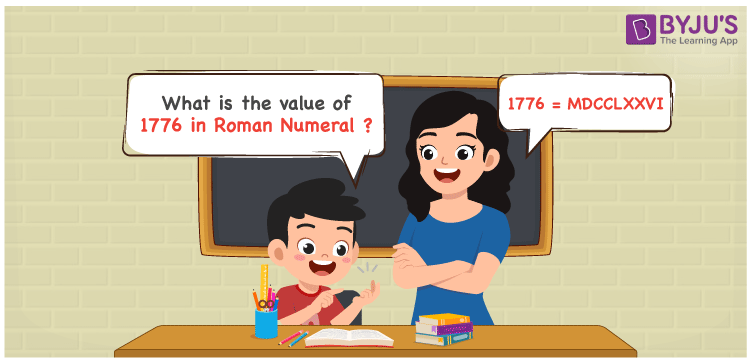# 1776 in Roman Numerals

1776 in Roman Numerals is MDCCLXXVI. The concept followed to convert 1776 to Roman Numerals can be grasped well by the students. The Roman numerals of 1776 are discussed in a crystal clear way so that students learn it without any queries. Hence, 1776 can be written as MDCCLXXVI in roman numerals.

 Number Roman Numeral 1776 MDCCLXXVI## How to Write 1776 in Roman Numerals?

The conversion of 1776 to MDCCLXXVI can be understood well with the help of the steps available here.

1776 = 1000 + 500 + 100 + 100 + 50 + 10 + 10 + 5 + 1

1776 = M + D + C + C + L + X + X + V + I

1776 = MDCCLXXVI

## Video Lesson on Roman Numerals## Frequently Asked Questions on 1776 in Roman Numerals

Q1

### How to write 1776 in Roman Numerals?

1776 in Roman Numerals is written as MDCCLXXVI.
Q2

### Find 2000 – 224.

We know that

2000 in Roman Numerals = MM

224 in Roman Numerals = CCXXIV

As 2000 – 224 = 1776

So 2000 – 224 is 1776 which is written as MDCCLXXVI.

Q3

### How to convert 1776 to roman numerals?

In Roman numerals,

1776 = 1000 + 500 + 100 + 100 + 50 + 10 + 10 + 5 + 1

1776 = M + D + C + C + L + X + X + V + I

1776 = MDCCLXXVI